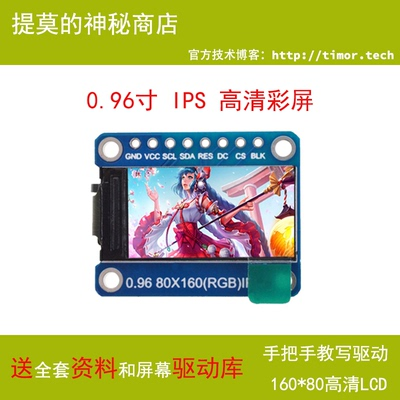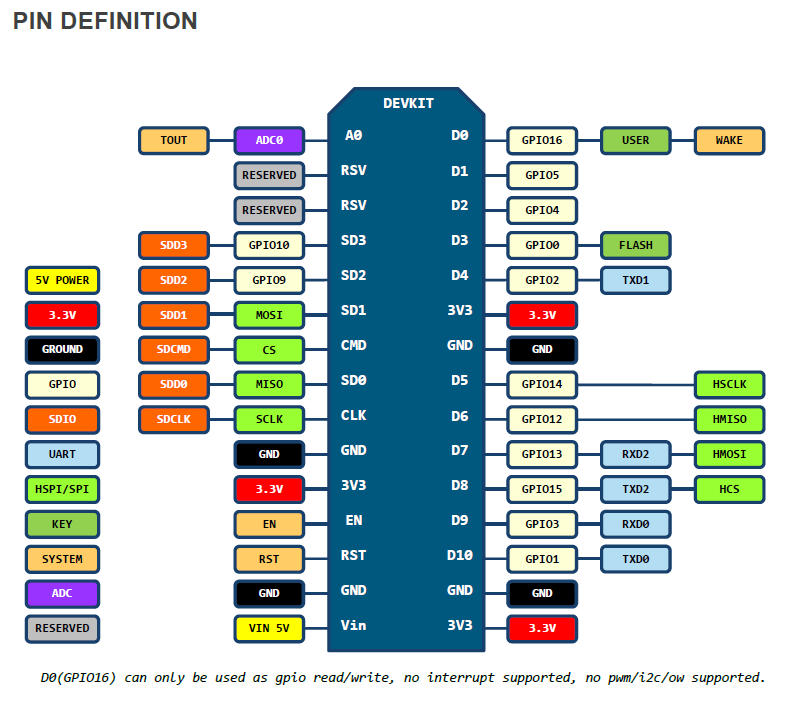# nodemcu 使用 Lua 编写/移植 ST7735S 0.96 寸彩色 LCD 屏幕驱动（SPI 通信）## 硬件接线## 移植到 nodemcu

``````-- Hardware SPI CLK  = GPIO14
-- Hardware SPI MOSI = GPIO13
-- Hardware SPI MISO = GPIO12 (not used)
cs  = 8 -- GPIO15, pull-down 10k to GND
dc  = 4 -- GPIO2
res = 0 -- GPIO16
bus = 1

node.setcpufreq(node.CPU160MHZ)

function spi_begin (id)
spi.setup(id, spi.MASTER, spi.CPOL_LOW, spi.CPHA_LOW, 8, 2)
-- we won't be using the HSPI /CS line, so disable it again
gpio.mode(cs, gpio.INPUT, gpio.PULLUP)
-- setup command and data pin.
gpio.mode(dc, gpio.OUTPUT)
gpio.mode(res, gpio.OUTPUT)
end

function spi_write (id, data)
spi.send(id, data)
end

function delay_ms (ms)
tmr.delay(ms * 1000)
end

function lcd_writeCmd(cmd)
gpio.write(dc, 0)
spi_write(bus, cmd)
end

function lcd_writeData (data)
gpio.write(dc, 1)
spi_write(bus, data)
end

function lcd_reset ()
gpio.write(res, 0)
delay_ms(100)
gpio.write(res, 1)
end

function lcd_init ()
lcd_reset()
lcd_writeCmd(0x11)
delay_ms(120)
lcd_writeCmd(0x21)

lcd_writeCmd(0xB1)
lcd_writeData(0x05)
lcd_writeData(0x3A)
lcd_writeData(0x3A)

lcd_writeCmd(0xB2)
lcd_writeData(0x05)
lcd_writeData(0x3A)
lcd_writeData(0x3A)

lcd_writeCmd(0xB3)
lcd_writeData(0x05)
lcd_writeData(0x3A)
lcd_writeData(0x3A)
lcd_writeData(0x05)
lcd_writeData(0x3A)
lcd_writeData(0x3A)

lcd_writeCmd(0xB4)
lcd_writeData(0x03)

lcd_writeCmd(0xC0)
lcd_writeData(0x62)
lcd_writeData(0x02)
lcd_writeData(0x04)

lcd_writeCmd(0xC1)
lcd_writeData(0xC0)

lcd_writeCmd(0xC2)
lcd_writeData(0x0D)
lcd_writeData(0x00)

lcd_writeCmd(0xC3)
lcd_writeData(0x8D)
lcd_writeData(0x6A)

lcd_writeCmd(0xC4)
lcd_writeData(0x8D)
lcd_writeData(0xEE)

lcd_writeCmd(0xC5)
lcd_writeData(0x0E)

lcd_writeCmd(0xE0)
lcd_writeData(0x10)
lcd_writeData(0x0E)
lcd_writeData(0x02)
lcd_writeData(0x03)
lcd_writeData(0x0E)
lcd_writeData(0x07)
lcd_writeData(0x02)
lcd_writeData(0x07)
lcd_writeData(0x0A)
lcd_writeData(0x12)
lcd_writeData(0x27)
lcd_writeData(0x37)
lcd_writeData(0x00)
lcd_writeData(0x0D)
lcd_writeData(0x0E)
lcd_writeData(0x10)

lcd_writeCmd(0xE1)
lcd_writeData(0x10)
lcd_writeData(0x0E)
lcd_writeData(0x03)
lcd_writeData(0x03)
lcd_writeData(0x0F)
lcd_writeData(0x06)
lcd_writeData(0x02)
lcd_writeData(0x08)
lcd_writeData(0x0A)
lcd_writeData(0x13)
lcd_writeData(0x26)
lcd_writeData(0x36)
lcd_writeData(0x00)
lcd_writeData(0x0D)
lcd_writeData(0x0E)
lcd_writeData(0x10)

lcd_writeCmd(0x3A)
lcd_writeData(0x05)

lcd_writeCmd(0x36)
lcd_writeData(0xC8)

lcd_writeCmd(0x29)
end

function lcd_setRegion(x1, y1, x2, y2)
lcd_writeCmd(0x2a)
lcd_writeData(0x00)
lcd_writeData(x1 + 0x1A)
lcd_writeData(0x00)
lcd_writeData(x2 + 0x1A)

lcd_writeCmd(0x2b)
lcd_writeData(0x00)
lcd_writeData(y1 + 1)
lcd_writeData(0x00)
lcd_writeData(y2 + 1)
lcd_writeCmd(0x2c)
end

function lcd_fill (color1, color2)
lcd_setRegion(0, 0, 79, 159)
for i=1,160 do
for j=1,80 do
lcd_writeData(color1)
lcd_writeData(color2)
end
tmr.wdclr()
end
end

function main()
spi_begin(bus)
lcd_init()

lcd_fill(0xf8, 0x00) -- red
lcd_fill(0x07, 0xe0) -- green
lcd_fill(0x00, 0x1f) -- blue
lcd_fill(0xff, 0xe0) -- yellow
lcd_fill(0xff, 0xff) -- white
end

main()
``````